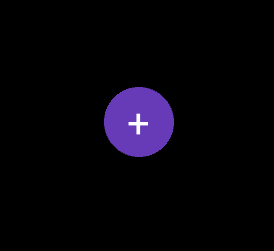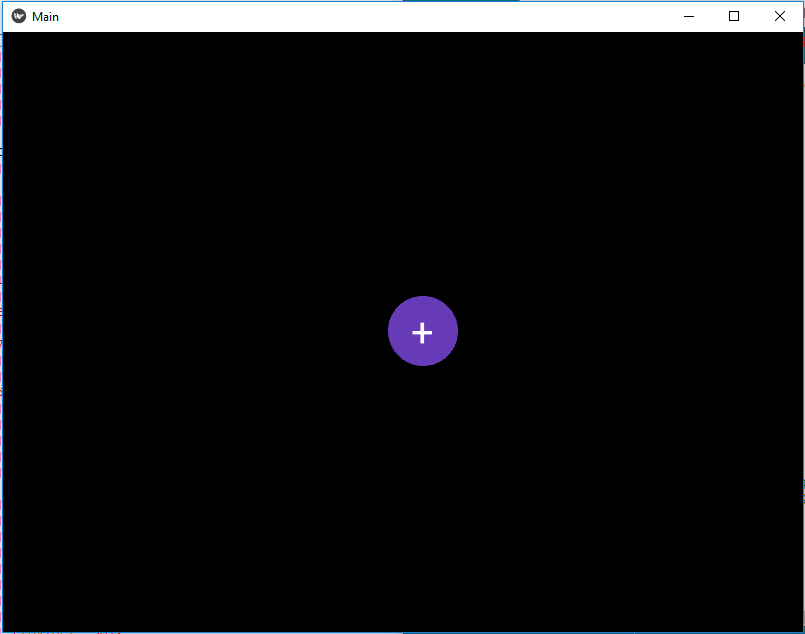# Animated Floating Action Button in kivy – Python

• Last Updated : 19 Oct, 2021

Kivy is a platform independent GUI tool in Python. As it can be run on Android, IOS, linux and Windows etc. It is basically used to develop the Android application, but it does not mean that it can not be used on Desktops applications.
In this article we will learn about how can we Add the Animation to a Floating Action button. To learn How to create it you must know about the Animation and Clock.

Animation : Animation and AnimationTransition are used to animate Widget properties. You must specify at least a property name and target value. To use an Animation, follow these steps:

Attention geek! Strengthen your foundations with the Python Programming Foundation Course and learn the basics.

To begin with, your interview preparations Enhance your Data Structures concepts with the Python DS Course. And to begin with your Machine Learning Journey, join the Machine Learning - Basic Level Course

• Setup an Animation object
• Use the Animation object on a Widget

To animate a Widget’s x or y position, simply specify the target x/y values where you want the widget positioned at the end of the animation:

```anim = Animation(x=100, y=100)
anim.start(widget)```

Clock: The Clock object allows you to schedule a function call in the future; once or repeatedly at specified intervals.
It is must to use kivy inbuilt module while working with Animation and clock –

```from kivy.animation import Animation
from kivy.clock import Clock```

```Basic Approach:

1) import kivy
2) import kivyApp
3) import Boxlayout
4) import Animation
5) Import Clock
6) Set minimum version(optional)
7) create Layout class and Add(create) animation in it
8) create App class
9) Set up .kv file :
2) Create Main Window
3) Add Float Button(don't forget to give id)
10) return Layout/widget/Class(according to requirement)
11) Run an instance of the class```

Kivy Tutorial – Learn Kivy with Examples.

Implementation of the Approach:
main.py file

## Python3

 `## Sample Python application demonstrating that``## How to create a button like floating Action Button``## in Kivy using .kv file``  ` `###################################################``# import modules``  ` `import` `kivy``  ` `# base Class of your App inherits from the App class.  ``# app:always refers to the instance of your application ``from` `kivy.app ``import` `App``  ` `# BoxLayout arranges widgets in either``# in a vertical fashion that``# is one on top of another or in a horizontal``# fashion that is one after another.``from` `kivy.uix.boxlayout ``import` `BoxLayout``  ` `# To change the kivy default settings ``# we use this module config ``from` `kivy.config ``import` `Config ``     ` `# 0 being off 1 being on as in true / false ``# you can use 0 or 1 && True or False ``Config.``set``(``'graphics'``, ``'resizable'``, ``True``)` `# The Clock object allows you to``# schedule a function call in the future``from` `kivy.clock ``import` `Clock` `# To work with Animation you must have to import it``from` `kivy.animation ``import` `Animation` `# creating the root widget used in .kv file``class` `MainWindow(BoxLayout):``    ``def` `__init__(``self``, ``*``*``kwargs):``        ``super``().__init__(``*``*``kwargs)` `        ``# Schedule the interval for the animation``        ``Clock.schedule_interval(``self``.breath, ``1``)` `    ``# Creating Animation function name breath``    ``def` `breath(``self``, dtx):` `        ``# create an animation object. This object could be stored``        ``# and reused each call or reused across different widgets.``        ``# += is a sequential step``        ``anim ``=` `(Animation(btn_size ``=``(``60``, ``60``), t ``=``'in_quad'``, duration ``=``.``5``)``+``               ``Animation(btn_size ``=``(``70``, ``70``), t ``=``'in_quad'``, duration ``=``.``5``))` `        ``# Call the button id``        ``tgt ``=` `self``.ids.cta` `        ``# Start the Animation``        ``anim.start(tgt)`  `# creating the App class in which name``#.kv file is to be named main.kv``class` `MainApp(App):``    ``# defining build()``    ``def` `build(``self``):``        ``# returning the instance of root class``        ``return` `MainWindow()` `# run the app``if` `__name__ ``=``=` `'__main__'``:``    ``MainApp().run()`

.kv file

## Python3

 `#.kv file implementation of BoxLayout`  `# using Float Layout for the creation of Floatbutton``# Here we are creating the properties of button``# Button will be created in Main window Box Layout` ```    ``id``: float_root  ``# Giving id to button``    ``size_hint: (``None``, ``None``)``    ``text: ''``    ``btn_size: (``70``, ``70``)``    ``size: (``70``, ``70``)``    ``bg_color: (``0.404``, ``0.227``, ``0.718``, ``1.0``)``    ``pos_hint: {``'x'``: .``6``}` `    ``# Adding shape and all, size, position to button``    ``Button:``        ``text: float_root.text``        ``markup: ``True``        ``font_size: ``40``        ``size_hint: (``None``, ``None``)``        ``size: float_root.btn_size``        ``pos_hint: {``'x'``: ``5.5``, ``'y'``: ``3.8``}``        ``background_normal: ''``        ``background_color: (``0``, ``0``, ``0``, ``0``)``        ``canvas.before:``            ``Color:``                ``rgba: (``0.404``, ``0.227``, ``0.718``, ``1.0``)``            ``Ellipse:``                ``size: ``self``.size``                ``pos: ``self``.pos``        ` `# Creation of main window``:``    ``BoxLayout:``        ``# Creating the Float button``        ``FloatButton:` `            ``# Giving id to the button``            ``# So that we can Apply the Animation``            ``id``: cta``            ``text: ``'+'``            ``markup: ``True``            ``background_color: ``1``, ``0``, ``1``, ``0`

Output:Video Output:

My Personal Notes arrow_drop_up